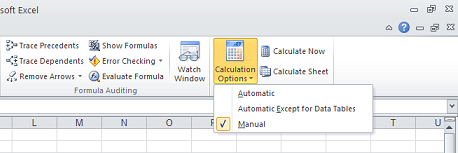# Setting Formula Calculation Mode of Workbook

Contents
[ ]

To set the formula calculation mode in Microsoft Excel:

1. Select Formulas and then Calculation Options.
2. Select one of the options.

Aspose.Cells also allows you to set the Formula Calculation Mode using the FormulaSettings.CalculationMode property. You can assign it the CalcModeType enumeration which has one of the following values:

The following sample code first creates a workbook, then it sets the formula calculation mode to Manual and saves the workbook as output Excel file on disk.

The output file. Note the formula calculation mode.